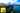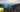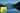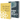# Linear Regression with Normal Equation in JavaScript

by Robin WieruchA recent article gave an introduction to the field of machine learning in JavaScript by predicting housing prices with gradient descent in a univariate regression problem. It used plain mathematical expressions and thus made use of the unvectorized implementation of gradient descent and the cost function. However, the unvectorized approach doesn't scale when applying it for multiple parameters (e.g. polynomial regression) or having a multivariate training set with multiple features n. That's the perfect point in time to use matrix operations for computational efficiency and thus to use the vectorized implementation of linear regression with gradient descent in univariate or multivariate training sets.

However, gradient descent is just one approach for a regression problem. There exists an alternative to gradient descent which is called normal equation when dealing with multivariate training sets. The following article will explain the normal equation in JavaScript and its advantages and disadvantages compared to gradient descent.

I highly recommend to take the Machine Learning course by Andrew Ng. This article will not explain the machine learning algorithms in detail, but only demonstrate their usage in JavaScript. The course on the other hand goes into detail and explains these algorithms in an amazing quality. At this point in time of writing the article, I learn about the topic myself and try to internalize my learnings by writing about them and applying them in JavaScript. If you find any parts for improvements, please reach out in the comments or create a Issue/Pull Request on GitHub.

## When to use Normal Equation over Gradient Descent

Normal equation for a regression problem is not a silver bullet. Compared to gradient descent it doesn’t need an iterative process to reduce the cost function over time. By explicitly taking the derivates, the function finds the optimum parameters for theta in only one mathematical expression. But why isn't it superior?

In a normal equation there isn't a learning rate alpha, there isn't a number of iterations and there aren't any improvements such as feature scaling. You can skip most of these improvements which you had to apply in gradient descent. The normal equation is still a vectorized matrix operation: `inv(X' * X) * X' * y`. That's everything speaking in favor for the normal equation over the iterative gradient descent. But, it turns out that the normal equation is slower compared to gradient descent when the number of features n goes up. In practice, when n exceeds 10,000 features, you can improve the computational efficiency by choosing an iterative algorithm such as gradient descent over the normal equation.

## Normal Equation in JavaScript

The following part will implement normal equation in JavaScript. The article will demonstrate it from scratch, but you will find later on the whole source code on GitHub for it. Before you can implement the algorithm, the training set needs to be prepared. Our starting point is the following function in JavaScript whereas the other parts will be implemented while reading the article:

`import math from 'mathjs';function init(matrix) {  let X = math.eval('matrix[:, 1:2]', {    matrix,  });  let y = math.eval('matrix[:, 3]', {    matrix,  });  let m = y.length;  // Part 1: Normal Equation}`

The function signature has access to the matrix as argument which includes all the information of the training set. Each row represents one house in the training set and each column represents one feature of the house. Thus each vector in the matrix represents a feature vector. By extracting X and y from the matrix as sub matrix and vector, there is on one side the matrix X with all the features that are used for the prediction (size, number of bedrooms) and on the other side y with the outcome (price) of it. Apart from that, m represents the size of the training set (number of houses).

Before implementing the normal equation in JavaScript, the matrix X needs to add an intercept term. Only this way the matrix operations work for theta and X. Again, I recommend to take the machine learning course by Andrew Ng to understand the intercept term in matrix X to perform the normal equation.

`import math from 'mathjs';function init(matrix) {  let X = math.eval('matrix[:, 1:2]', {    matrix,  });  let y = math.eval('matrix[:, 3]', {    matrix,  });  let m = y.length;  // Part 1: Normal Equation  X = math.concat(math.ones([m, 1]).valueOf(), X);  let theta = normalEquation(X, y);}function normalEquation(X, y) {  ...  return theta;}`

Now there comes the part of implementing the normal equation in JavaScript. You will be surprised that it isn't too much code, because it is only one mathematical expression that was already mentioned before.

`function normalEquation(X, y) {  let theta = math.eval(`inv(X' * X) * X' * y`, {    X,    y,  });  return theta;}`

That's already it to compute theta with a normal equation. Now you can predict further housing prices based on your trained hypothesis.

`function init(matrix) {  ...  // Part 2: Predict Price of 1650 square meter and 3 bedroom house  let houseVector = [1, 1650, 3];  let price = math.eval('houseVector * theta', {    houseVector,    theta,  });  console.log('Predicted price for a 1650 square meter and 3 bedroom house: ', price);}`

Finally you can find the whole source code in this GitHub repository. If you liked it, make sure to star it.

Hopefully the article was helpful to understand the differences between gradient descent and normal equation for a regression problem and to implement normal equation in JavaScript for a practical use case. If you have any suggestions for improvements, please comment below.## Gradient Descent with Vectorization in JavaScript

A recent article gave an introduction to the field of machine learning in JavaScript by predicting housing prices with gradient descent in a univariate regression problem. It used plain mathematical…## Multivariate Linear Regression, Gradient Descent in JavaScript

A recent article gave an introduction to the field of machine learning in JavaScript by predicting housing prices with gradient descent in a univariate regression problem. It used plain mathematical…